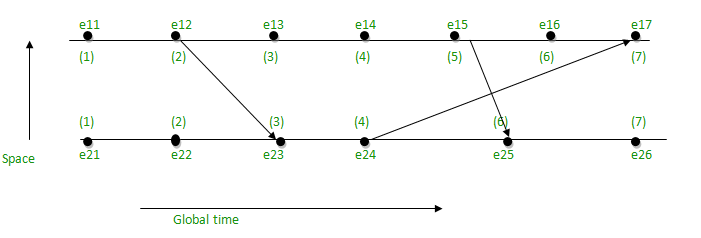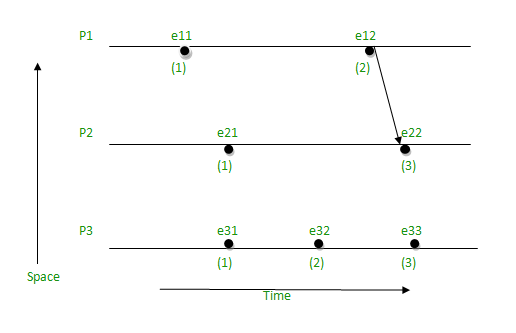# Lamport’s logical clock

• Difficulty Level : Hard
• Last Updated : 24 Nov, 2021

Lamport’s Logical Clock was created by Leslie Lamport. It is a procedure to determine the order of events occurring. It provides a basis for the more advanced Vector Clock Algorithm. Due to the absence of a Global Clock in a Distributed Operating System Lamport Logical Clock is needed.

Algorithm:

• Happened before relation(->): a -> b, means ‘a’ happened before ‘b’.
• Logical Clock: The criteria for the logical clocks are:
• [C1]: Ci (a) < Ci(b), [ Ci -> Logical Clock, If ‘a’ happened before ‘b’, then time of ‘a’ will be less than ‘b’ in a particular process. ]
• [C2]: Ci(a) < Cj(b), [ Clock value of Ci(a) is less than Cj(b) ]

Reference:

• Process: Pi
• Event: Eij, where i is the process in number and j: jth event in the ith process.
• tm: vector time span for message m.
• Ci vector clock associated with process Pi, the jth element is Ci[j] and contains Pi‘s latest value for the current time in process Pj.
• d: drift time, generally d is 1.

Implementation Rules[IR]:

• [IR1]: If a -> b [‘a’ happened before ‘b’ within the same process] then, Ci(b)  =Ci(a) + d
• [IR2]: Cj = max(Cj, tm + d) [If there’s more number of processes, then tm = value of Ci(a), Cj = max value between Cj and tm + d]

For Example:• Take the starting value as 1, since it is the 1st event and there is no incoming value at the starting point:
• e11 = 1
• e21 = 1
• The value of the next point will go on increasing by d (d = 1), if there is no incoming value i.e., to follow [IR1].
• e12 = e11 + d = 1 + 1 = 2
• e13 = e12 + d = 2 + 1 = 3
• e14 = e13 + d = 3 + 1 = 4
• e15 = e14 + d = 4 + 1 = 5
• e16 = e15 + d = 5 + 1 = 6
• e22 = e21 + d = 1 + 1 = 2
• e24 = e23 + d = 3 + 1 = 4
• e26 = e25 + d = 6 + 1 = 7
• When there will be incoming value, then follow [IR2] i.e., take the maximum value between Cj and Tm + d.
• e17 = max(7, 5) = 7, [e16 + d = 6 + 1 = 7, e24 + d = 4 + 1 = 5, maximum among 7 and 5 is 7]
• e23 = max(3, 3) = 3, [e22 + d = 2 + 1 = 3, e12 + d = 2 + 1 = 3, maximum among 3 and 3 is 3]
• e25 = max(5, 6) = 6, [e24 + 1 = 4 + 1 = 5, e15 + d = 5 + 1 = 6, maximum among 5 and 6 is 6]

Limitation:

• In case of [IR1], if a -> b, then C(a) < C(b) -> true.
• In case of [IR2], if  a -> b, then C(a) < C(b) -> May be true or may not be true.Below is the C program to implement Lamport’s Logical Clock:

## C++

 `// C++ program to illustrate the Lamport's``// Logical Clock` `#include ``using` `namespace` `std;` `// Function to find the maximum timestamp``// between 2 events``int` `max1(``int` `a, ``int` `b)``{``    ``// Return the greatest of th two``    ``if` `(a > b)``        ``return` `a;``    ``else``        ``return` `b;``}` `// Function to display the logical timestamp``void` `display(``int` `e1, ``int` `e2,``             ``int` `p1, ``int` `p2)``{``    ``int` `i;` `    ``cout << ``"\nThe time stamps of "``         ``"events in P1:\n"``;` `    ``for` `(i = 0; i < e1; i++) {``        ``cout << p1[i] << ``" "``;``    ``}` `    ``cout << ``"\nThe time stamps of "``         ``"events in P2:\n"``;` `    ``// Print the array p2[]``    ``for` `(i = 0; i < e2; i++)``        ``cout << p2[i] << ``" "``;``}` `// Function to find the timestamp of events``void` `lamportLogicalClock(``int` `e1, ``int` `e2,``                         ``int` `m)``{``    ``int` `i, j, k, p1[e1], p2[e2];` `    ``// Initialize p1[] and p2[]``    ``for` `(i = 0; i < e1; i++)``        ``p1[i] = i + 1;` `    ``for` `(i = 0; i < e2; i++)``        ``p2[i] = i + 1;``    ``cout << ``"\t"``;``    ``for` `(i = 0; i < e2; i++)``        ``cout << ``"\te2"` `<< i + 1;` `    ``for` `(i = 0; i < e1; i++) {` `        ``cout << ``"\n e1"` `<< i + 1 << ``"\t"``;` `        ``for` `(j = 0; j < e2; j++)``            ``cout << m[i][j] << ``"\t"``;``    ``}` `    ``for` `(i = 0; i < e1; i++) {``        ``for` `(j = 0; j < e2; j++) {` `            ``// Change the timestamp if the``            ``// message is sent``            ``if` `(m[i][j] == 1) {``                ``p2[j] = max1(p2[j], p1[i] + 1);``                ``for` `(k = j + 1; k < e2; k++)``                    ``p2[k] = p2[k - 1] + 1;``            ``}` `            ``// Change the timestamp if the``            ``// message is received``            ``if` `(m[i][j] == -1) {``                ``p1[i] = max1(p1[i], p2[j] + 1);``                ``for` `(k = i + 1; k < e1; k++)``                    ``p1[k] = p1[k - 1] + 1;``            ``}``        ``}``    ``}` `    ``// Function Call``    ``display(e1, e2, p1, p2);``}` `// Driver Code``int` `main()``{``    ``int` `e1 = 5, e2 = 3, m;` `    ``// message is sent and received``    ``// between two process` `    ``/*dep[i][j] = 1, if message is sent``                   ``from ei to ej``    ``dep[i][j] = -1, if message is received``                    ``by ei from ej``    ``dep[i][j] = 0, otherwise*/``    ``m = 0;``    ``m = 0;``    ``m = 0;``    ``m = 0;``    ``m = 0;``    ``m = 1;``    ``m = 0;``    ``m = 0;``    ``m = 0;``    ``m = 0;``    ``m = 0;``    ``m = 0;``    ``m = 0;``    ``m = -1;``    ``m = 0;` `    ``// Function Call``    ``lamportLogicalClock(e1, e2, m);` `    ``return` `0;``}`

## C

 `// C program to illustrate the Lamport's``// Logical Clock``#include ` `// Function to find the maximum timestamp``// between 2 events``int` `max1(``int` `a, ``int` `b)``{``    ``// Return the greatest of th two``    ``if` `(a > b)``        ``return` `a;``    ``else``        ``return` `b;``}` `// Function to display the logical timestamp``void` `display(``int` `e1, ``int` `e2,``             ``int` `p1, ``int` `p2)``{``    ``int` `i;` `    ``printf``(``"\nThe time stamps of "``           ``"events in P1:\n"``);` `    ``for` `(i = 0; i < e1; i++) {``        ``printf``(``"%d "``, p1[i]);``    ``}` `    ``printf``(``"\nThe time stamps of "``           ``"events in P2:\n"``);` `    ``// Print the array p2[]``    ``for` `(i = 0; i < e2; i++)``        ``printf``(``"%d "``, p2[i]);``}` `// Function to find the timestamp of events``void` `lamportLogicalClock(``int` `e1, ``int` `e2,``                         ``int` `m)``{``    ``int` `i, j, k, p1[e1], p2[e2];` `    ``// Initialize p1[] and p2[]``    ``for` `(i = 0; i < e1; i++)``        ``p1[i] = i + 1;` `    ``for` `(i = 0; i < e2; i++)``        ``p2[i] = i + 1;` `    ``for` `(i = 0; i < e2; i++)``        ``printf``(``"\te2%d"``, i + 1);` `    ``for` `(i = 0; i < e1; i++) {` `        ``printf``(``"\n e1%d \t"``, i + 1);` `        ``for` `(j = 0; j < e2; j++)``            ``printf``(``"%d\t"``, m[i][j]);``    ``}` `    ``for` `(i = 0; i < e1; i++) {``        ``for` `(j = 0; j < e2; j++) {` `            ``// Change the timestamp if the``            ``// message is sent``            ``if` `(m[i][j] == 1) {``                ``p2[j] = max1(p2[j], p1[i] + 1);``                ``for` `(k = j + 1; k < e2; k++)``                    ``p2[k] = p2[k - 1] + 1;``            ``}` `            ``// Change the timestamp if the``            ``// message is received``            ``if` `(m[i][j] == -1) {``                ``p1[i] = max1(p1[i], p2[j] + 1);``                ``for` `(k = i + 1; k < e1; k++)``                    ``p1[k] = p1[k - 1] + 1;``            ``}``        ``}``    ``}` `    ``// Function Call``    ``display(e1, e2, p1, p2);``}` `// Driver Code``int` `main()``{``    ``int` `e1 = 5, e2 = 3, m;` `    ``// message is sent and received``    ``// between two process` `    ``/*dep[i][j] = 1, if message is sent``                   ``from ei to ej``    ``dep[i][j] = -1, if message is received``                    ``by ei from ej``    ``dep[i][j] = 0, otherwise*/``    ``m = 0;``    ``m = 0;``    ``m = 0;``    ``m = 0;``    ``m = 0;``    ``m = 1;``    ``m = 0;``    ``m = 0;``    ``m = 0;``    ``m = 0;``    ``m = 0;``    ``m = 0;``    ``m = 0;``    ``m = -1;``    ``m = 0;` `    ``// Function Call``    ``lamportLogicalClock(e1, e2, m);` `    ``return` `0;``}`

## Python3

 `# Python program to illustrate the Lamport's``# Logical Clock` `# Function to find the maximum timestamp``# between 2 events``def` `max1(a, b) :` `    ``# Return the greatest of th two``    ``if` `a > b :``        ``return` `a``    ``else` `:``        ``return` `b` `# Function to display the logical timestamp``def` `display(e1, e2, p1, p2) :``    ``print``()``    ``print``(``"The time stamps of events in P1:"``)``    ``for` `i ``in` `range``(``0``, e1) :``        ``print``(p1[i], end ``=` `" "``)``    ` `    ``print``()``    ``print``(``"The time stamps of events in P2:"``)``    ` `    ``# Print the array p2[]``    ``for` `i ``in` `range``(``0``, e2) :``        ``print``(p2[i], end ``=` `" "``)` `# Function to find the timestamp of events``def` `lamportLogicalClock(e1, e2, m) :``    ``p1 ``=` `[``0``]``*``e1``    ``p2 ``=` `[``0``]``*``e2` `    ``# Initialize p1[] and p2[]``    ``for` `i ``in` `range` `(``0``, e1) :``        ``p1[i] ``=` `i ``+` `1` `    ``for` `i ``in` `range``(``0``, e2) :``        ``p2[i] ``=` `i ``+` `1``    ` `    ``for` `i ``in` `range``(``0``, e2) :``        ``print``(end ``=` `'\t'``)``        ``print``(``"e2"``, end ``=` `"")``        ``print``(i ``+` `1``, end ``=` `"")``    ` `    ``for` `i ``in` `range``(``0``, e1) :``        ``print``()``        ``print``(``"e1"``, end ``=` `"")``        ``print``(i ``+` `1``, end ``=` `"\t"``)` `        ``for` `j ``in` `range``(``0``, e2) :``            ``print``(m[i][j], end ``=` `"\t"``)``        ` `    ``for` `i ``in` `range``(``0``, e1) :` `        ``for` `j ``in` `range``(``0``, e2) :``          ` `            ``# Change the timestamp if the``            ``# message is sent``            ``if``(m[i][j] ``=``=` `1``) :``                ``p2[j] ``=` `max1(p2[j], p1[i] ``+` `1``)``                ``for` `i ``in` `range``(j ``+` `1``, e2) :``                    ``p2[k] ``=` `p2[k ``-` `1``] ``+` `1` `            ``# Change the timestamp if the``            ``# message is received``            ``if``(m[i][j] ``=``=` `-``1``) :``                ``p1[i] ``=` `max1(p1[i], p2[j] ``+` `1``)``                ``for` `k ``in` `range``(i ``+` `1``, e1) :``                    ``p1[k] ``=` `p1[k ``-` `1``] ``+` `1` `    ``# Function Call``    ``display(e1, e2, p1, p2)` `# Driver Code` `if` `__name__ ``=``=` `"__main__"` `:``    ``e1 ``=` `5``    ``e2 ``=` `3``    ``m ``=` `[[``0``]``*``3` `for` `i ``in` `range``(``0``,``5``)]``    ` `    ``# dep[i][j] = 1, if message is sent``    ``# from ei to ej``    ``# dep[i][j] = -1, if message is received``    ``# by ei from ej``    ``# dep[i][j] = 0, otherwise` `    ``m[``0``][``0``] ``=` `0``    ``m[``0``][``1``] ``=` `0``    ``m[``0``][``2``] ``=` `0``    ``m[``1``][``0``] ``=` `0``    ``m[``1``][``1``] ``=` `0``    ``m[``1``][``2``] ``=` `1``    ``m[``2``][``0``] ``=` `0``    ``m[``2``][``1``] ``=` `0``    ``m[``2``][``2``] ``=` `0``    ``m[``3``][``0``] ``=` `0``    ``m[``3``][``1``] ``=` `0``    ``m[``3``][``2``] ``=` `0``    ``m[``4``][``0``] ``=` `0``    ``m[``4``][``1``] ``=` `-``1``    ``m[``4``][``2``] ``=` `0``    ` `    ``# Function Call``    ``lamportLogicalClock(e1, e2, m)` `    ``# This code is contributed by rakeshsahni`

## Javascript

 ``
Output
```        e21    e22    e23
e11    0    0    0
e12    0    0    1
e13    0    0    0
e14    0    0    0
e15    0    -1    0
The time stamps of events in P1:
1 2 3 4 5
The time stamps of events in P2:
1 2 3 ```

Time Complexity: O(e1 * e2 * (e1 + e2))
Auxiliary Space: O(e1 + e2)

My Personal Notes arrow_drop_up